Next: About this document ... Up: lab_template Previous: lab_template

Subsections

# The exponential and logarithmic functions

## Exponential growth

The simple model for growth is exponential growth, where it is assumed that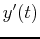is proportional to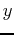. That is,Separating the variables and integrating, we haveso that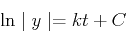In the case of exponential growth, we can drop the absolute value signs around, becausewill always be a positive quantity. Solving for, we obtain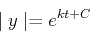which we may write in the form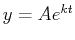, where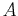is an arbitrary positive constant. The same formula is used for exponential decay, except the decay constant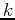is negative.

### Newton's law of cooling

What is usually called Newton's law of cooling is a simple model for the change in temperature of an object that is in contact with an environment at a different temperature. It says that the rate of change of the temperature of an object is proportional to the difference between the object's temperature and the temperature of the environment. Mathematically, this can be expressed as the differential equation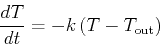whereis the constant of proportionality and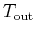is the temperature of the environment. Using a technique called separation of variables it isn't hard to derive the solution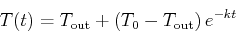where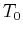is the temperature of the object at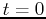.

## Inverse Functions

Consider the functionsdefined byTo be able to get an inverse the function must be one-to-one. You can plot the functions to get a hint as to whether they are invertible or not.
> f:=x->exp(x)+exp(-x);
> plot(f(x),x=-5..5);
> g:=x->exp(x)-exp(-x);
> plot(g(x),x=-5..5);

Both satisfy the vertical-line test but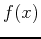is not invertible since it does not satisfy the horizontal-line test. Indeed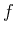is not one-to-one, for instance. From the plot it seems that the function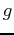is one-to-one. In order to determine its inverse we solve for x.
> solve(g(x)=y,x);

We observe that one of the solutions is not defined since the argument of the logarithm can only be positive. Thus: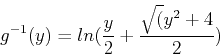> ginv:=y->ln(y/2+sqrt(y^2+4)/2);

Let's look at the plot along with the line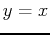to see if our functions seem to make sense.
> plot({x,g(x),ginv(x)},x=-20..20,y=-20..20,scaling=constrained);

Let's check that we have computed the right inverse. By definition the composot ion of the functions should be the linesince an inverse is the reflectio n about this line.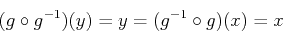> g(ginv(y));
> simplify(%);
> ginv(g(x));
> simplify(%);

We are having difficulty gettingfor the last composition. Think about what issue the computer has in dealing with this simplification as you will come across this in the exercises.

## Exercises

1. A 5 lb. roast having initial temperature of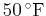is put into a pre-heated oven at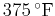. How much longer will it take for the roast to be medium rare (a temperature ofif the roast isafter 75 minutes.

2. The loudness of sound is measured in decibels in honor of Alexander Graham Bell (1847-1922), inventor of the telephone. If the variation in pressure is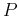pounds per square inch, then the loudness in decibels is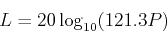Find how many times greater is the variation in pressure caused by a rock band at 115 decibels than a soft rock concert at 85 decibels.

3. For each function below, use the background example under the section titled Inverse Functions to help answer each of the following. Be sure to shrink your graphs.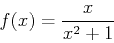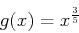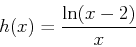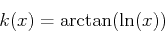A)
Plot each funtion over a range that gives a good representation of the function. Which functions are not invertible and why?
B)
Find the inverse of the invertible function(s).
C)
For at least one of the invertivle functions, plot the function and its inverse along with the line. Use a domain that shows that the functions are inverses.
D)
Show that you have the correct inverse(s) in part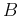by using the composite definition. (If you come across a simplifying problem type the command assume(x>0).)Next: About this document ... Up: lab_template Previous: lab_template
Dina J. Solitro-Rassias
2014-02-24